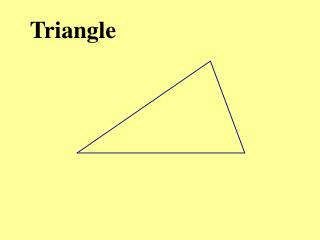DownloadDownload PresentationTriangle

# Triangle

Télécharger la présentation## Triangle

- - - - - - - - - - - - - - - - - - - - - - - - - - - E N D - - - - - - - - - - - - - - - - - - - - - - - - - - -
##### Presentation Transcript

1. Triangle

2. Ratio of the area of triangles Theorem 1

3. Example In the figure, BC// DE, AC= 3 cm and CE= 4 cm. Find

4. Class work In the figure, PSQ, QXR and RYP are straight lines. If the area of is , find the area of the parallelogram SXRY.

5. 81 36 Area of parallelogram = 225 – 81 - 36 = 108 Area of parallelogram is

6. Triangle

7. Triangle C height A B base

8. Triangle

9. Triangle base height

10. Triangle

11. Triangle base height

12. Area of Triangle C height A B base

13. What is the relationship between the heights of and ?

14. Triangles have common height h

15. For triangles with commonheight, Theorem 2

16. Eg.3) Given that BC : DC = 5: 1 Find and have common height,

17. Eg.3) Given that BC : DC = 5: 1 Find and have common height, = 4

18. Eg.4) Given that AD = 3 cm and CD = 1 cm. Find and have common height,

19. Class work 5.) In the figure, find the area of : area of .

20. Class work 5.) In the figure, find the area of : area of .

21. Class work 5.) In the figure, find the area of : area of . = 1

22. 6.) In the figure, given that QX:XR = 5:6 and PY:YR =5:3 calculate the area of (a) (b)

23. 6.) In the figure, given that QX:XR = 5:6 and PY:YR =5:3 5x 6x

24. 6.) In the figure, given that QX:XR = 5:6 and PY:YR =5:3 5x 6x

25. 6.) In the figure, given that QX:XR = 5:6 and PY:YR =5:3 5y 3y 5x 6x

26. 6.) In the figure, given that QX:XR = 5:6 and PY:YR =5:3 5y 3y 5x 6x

27. 7) In the figure, PQRS is a rectangle. M is a midpoint of QR. PR and MS intersects at N. Find the area of : area of PQMN.

28. In the figure, PQRS is a rectangle. M is a midpoint of QR. PR and MS intersects at N. Find the area of : area of PQMN.

29. In the figure, PQRS is a rectangle. M is a midpoint of QR. PR and MS intersects at N. Find the area of : area of PQMN.

30. Find the area of : area of PQMN. 4A 2y 2A y A Considering They have the common height.

31. Find the area of : area of PQMN. 4A 2y 2A y A Hence, = 2A : 5A = 2 : 5

32. 8.) In the figure, PX:XQ = 1: 2, PY:YR = 3:2. Area of : Area of = ?

33. In the figure, PX:XQ = 1: 2, PY:YR = 3:2. Area of : Area of = ? and have the common height

34. In the figure, PX:XQ = 1: 2, PY:YR = 3:2. Area of : Area of = ? and have the common height

35. In the figure, PX:XQ = 1: 2, PY:YR = 3:2. Area of : Area of = ? and have the common height = 2A : 5A = 2 : 5

36. 9.) In the figure, PQRS is a rectangle. RSX is a straight line and PX// QS. If the area of PQRS is 24 and Y is a point on QR such that QY :YR = 3:1, find the area of .

37. 10.) In the figure, if , Find

38. 10.) In the figure, if , A 3A

39. 10.) In the figure, if , A x 3A 3x

40. 10.) In the figure, if , A x 3A 3x 9A

41. 10.) In the figure, if , A x 3A 3A 3x 9A

42. 10.) In the figure, if , A x 3A 3A 3x 9A

43. Ratio of the area of similar triangles Theorem 1

44. For triangles with commonheight, Theorem 2